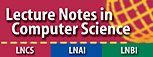### Workshop Goals

This workshop is a forum of mathematicians, computer scientists, engineers and physicists performing research on finite field arithmetic, interested in communicating the advances in the theory, applications, and implementations of finite fields. The workshop will help to bridge the gap between the mathematical theory of finite fields and their hardware/software implementations and technical applications.

### Main Workshop Themes

The topics of WAIFI 2022 include but are not limited to:

• Theory of finite field arithmetic:

• Bases (normal bases, duality, complexity ...)
• Polynomial factorization, irreducible polynomials
• Primitive polynomials, permutation polynomials
• Special functions over finite fields (Boolean functions, APN functions, ...)
• Curves over finite fields
• Algebraic dynamical systems over finite fields

• Hardware/Software implementation of finite field arithmetic:

• Optimal arithmetic modules
• Design and implementation of finite field arithmetic processors
• Design and implementation of arithmetic algorithms
• Pseudorandom number generators
• Hardware/Software Co-design
• IP (Intellectual Property) components
• Field programmable and reconfigurable systems

• Applications:

• Cryptography
• Communication systems
• Error correcting codes
• Finite geometry
• Quantum computing

### Proceedings

The proceedings will be published in the Springer Lecture Notes in Computer Science series after the workshop as post-proceedings.

 LNCS: Springer Lecture Notes in Computer Science.